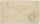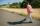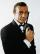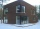Sisters

Sisters Hanka and Vera goes mostly tograndmother by bicycle. Journey took Hanka 30 minutes and Vera 20 minutes. How long will Vera catch up with Hanka when started from home five minutes later than Hanka?

Result

t =  10 min

Solution:(t+5)/30 = t/20

t = 10

t = 10

Calculated by our simple equation calculator.

Leave us a comment of this math problem and its solution (i.e. if it is still somewhat unclear...):Be the first to comment!To solve this verbal math problem are needed these knowledge from mathematics:

Do you have a linear equation or system of equations and looking for its solution? Or do you have quadratic equation? Do you want to convert length units?

Next similar math problems:

1. Forest nurseryIn the forest nursery after winter, they found that 1/10 stems died out of them. For them, they land 193 new spruces. How many spruces are in the forest nursery?
2. Six yearsIn six years Jan will be twice as old as he was six years ago. How old is he?
3. Cars 6At 9:00 am two cars started from the same town and traveled at a rate of 35 miles per hour and the other car traveled at a rate of 40 miles per hour. After how many hours will the cars be 30 miles apart?
4. AuntAnnie aunt is 35 years old, Annie is 14 years. For how many years will aunt have twice as many years as Annie?
5. NormThree workers planted 3555 seedlings of tomatoes in one dey. First worked at the standard norm, the second planted 120 seedlings more and the third 135 seedlings more than the first worker. How many seedlings were standard norm?
6. Unknown number 11That number increased by three equals three times itself?
7. Round-tripA woman works at a law firm in city A which is about 50 miles from city B. She must go to the law library in city B to get a document. Find how long it takes her to drive​ round-trip if she averages 40 mph.
8. One-thirdA one-third of unknown number is equal to five times as great as the difference of the same unknown number and number 28. Determine the unknown number.
9. PedestrianDana started at 10:00 from the point A to point B. These points are distant 12 km. Determine how fast Daniel went when the place B arrived at 11:54. Speed express in km/h.
10. Simple equation 9Solve the following equation: -8y+5=-9y+9
11. Bed timeTiffany was 5 years old; her week night bedtime grew by ¼ hour each year. If, at age 18, her curfew time is 11pm, what was her bed time when she was 5 years old?
12. Simple equationSolve the following simple equation: 2. (4x + 3) = 2-5. (1-x)
13. ChasePaul travel at 60 km/h. After 18 km ride found that he forgot an important book. His brother Thomas behind him carrying it. How fast must Thomas go to catch up with Paul in 31 minutes?
14. Equation?
15. Bob traveledBob traveled 20 meters to Dave's house. He arrived in 3 minutes. What was his average speed for the trip.
16. Cyclist 9A cyclist travels at a a speed of 4.25 km per hour. At that rate, how far can he travel in 3.75 hours?
17. Simple equation 8Solve the following equation: 36=-(1+7x)-6(-7-x)